Nhảy đến nội dung
x

## Division of Computational Mathematics and Engineering

1. Introduction

Division of Computational Mathematics and Engineering (CME) was established in 2013 as one of independent research groups of Ton Duc Thang University. CME has been then upgraded into the first division of the Institute for Computational Science (INCOS) in 2014, has developed fast as one of the most dynamic and effective research centers about computational science in Ho Chi Minh City. So far, CME has attracted many young and talented researchers in various disciplines related to computational science.

2. Mission and vision

- Develop advanced computational mathematics and engineering for solving complex problems in engineering and science and to provide optimal computational solutions for current and future industries.

- Establish itself as a typical research division of excellence in Vietnam and a reputable R&D center of excellence for advanced computational mathematics and engineering both regionally and internationally.

3. Research topics

3.1. Numerical methods

• Smoothed Finite Element Methods (S-FEM);

• Isogeometric Analysis (IGA).

3.2. Computational mechanics

• Computational composite plate/shell mechanics (analyze using S-FEM và IGA; analyze using FSDT, HSDT, C0-HSDT, layer-wise, unified theory; analyze using multi-layers composite materials, functionally graded materials (FGM), piezoelectricity integratedmaterial …);

• Computational fracture solid mechanics (analyze using Enriched S-FEM, analyze using Enriched IGA; analyze using extended S-FEM);

• Computational nonlinear mechanics (material nonlinear (plasticity), geometric nonlinear (large deformation (plates, shells)));

• Computational Mechanics in Multiple physical environments (Analyze fluid – solid interaction problems; analyze plates on foundations under moving loads; analyze plate/shell using the piezoelectricity integrated materials subjected to both mechanical and electrical loads;  analyze plate/shell using Functionally Graded Materials).

3.3. Computational optimization

• Topology optimization;

• Structural optimization;

• Intelligent optimization;

• Multi-objective optimization;

• Reliability-based design optimization;

• Dynamic optimization;

• Limit analysis of foundation, plates, and shells.

3.4. Probability, statistics and reliability analysis

• Data mining;

• Reliability analysis of structures;

• Intelligent reliability analysis.

3.5. Computational and analysis mathematics

• Stochastic differential equations, optimization and optimization stochastic control;

• Set-valued differential equations;

• Fuzzy differential equations, interval-valued differential equations and their applications;

• Delay differential equations and their applications;

• Operator and trace inequalities - matrix analysis - non-commutative measure and integral theory - quantum information theory;

• Topological methods in nonlinear functional analysis - set-valued analysis - differential inclusions and functional differential equations - bifurcation theory - differential variational inequalities.

3.6. Computational micro and nanotechnology (CMN)

• Modeling and simulation of microsystems;

• Modeling and simulation of nanomaterials and nanodevices;

• Antenna and wireless communication - Terahertz Opto-electronics – metamaterials.

4. Current membersDr. Nguyen Thoi Trung, Prof Positions: • Research Professor of Ton Duc Thang University, Vietnam • Director of Institute for Computational Science (INCOS), Ton Duc Thang University, Vietnam Areas of expertise: Numerical methods, computational mechanics, computational optimization, reliability analysis, artificial intelligence, health structural monitoring Editorship: • Editor Board: Mathematical Problems in Engineering (2011 - 2014) • Deputy Editor-in-Chief of Journal of Advanced Engineering and Computation (2016 - now) Research track record (until December of 2017): • ISI papers: 105 • Total ISI Citations: 3761 • ISI H-index (Scopus): 34 • Top ISI journals (at most 5): 1. Computational Mechanics 2. International journal for numerical methods in Engineering 3. Computer methods in Applied Mechanics and Engineering 4. Smart Materials and Structures 5. Composites Part B: EngineeringDang Trung Hau, Ph.D. candidate Position: Member of Division of Computational Mathematics and Engineering (CME), Institute for Computational Science (INCOS), Ton Duc Thang University, Ho Chi Minh City, Vietnam Areas of expertise: FEM for plate and shell, modeling smart materialVo Duy Trung, Ph.D. candidate Position: Member of Division of Computational Mathematics and Engineering (CME), Institute for Computational Science (INCOS), Ton Duc Thang University, Ho Chi Minh City, Vietnam Areas of expertise: Numerical analysis, applied mathematics, modeling and numerical simulations, high performance computing, health structural monitoringHo Huu Vinh, Ph.D. candidate Position: Member of Division of Computational Mathematics and Engineering (CME), Institute for Computational Science (INCOS), Ton Duc Thang University, Vietnam Areas of expertise: Computational intelligent, numerical methods, structural reliability assessment, a reliability based design optimization for structuresNguyen Trang Thao, Ph.D. candidate Position: Member of Division of Computational Mathematics and Engineering (CME), Institute for Computational Science (INCOS), Ton Duc Thang University, Ho Chi Minh City, Vietnam Areas of expertise: Probability and statistics, data mining (clustering classification)Ngo Van Hoa, BSc Position: Member of Division of Computational Mathematics and Engineering (CME), Institute for Computational Science (INCOS), Ton Duc Thang University, Ho Chi Minh City, Vietnam Areas of expertise: Fuzzy analysis, fuzzy set and systems, control theory, computer science, artificial intelligence, computer-aided engineeringHo Kieu Diem, MSc Position: Member of Division of Computational Mathematics and Engineering (CME), Institute for Computational Science (INCOS), Ton Duc Thang University, Ho Chi Minh City, Vietnam Areas of expertise: Statistics: Data mining (Clustering, Classification), Apply Statistics in Economic, Technical Analysis, Social SciencesDr. Truong Khac Tung Position: Member of Division of Computational Mathematics and Engineering (CME), Institute for Computational Science (INCOS), Ton Duc Thang University, Ho Chi Minh City, Vietnam Areas of expertise: Artificial intelligence, optimization algorithms, high performance computing (HPC)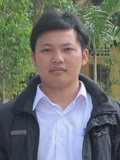Dr. Dinh Trung Hoa Position: Member of Division of Computational Mathematics and Engineering (CME), Institute for Computational Science (INCOS), Ton Duc Thang University, Ho Chi Minh City, Vietnam Areas of expertise: Operator and trace inequalities, matrix analysis, non-commutative measure and integral theory, quantum information theoryDr. Nguyen Van Loi Position: Member of Division of Computational Mathematics and Engineering (CME), Institute for Computational Science (INCOS), Ton Duc Thang University, Ho Chi Minh City, Vietnam Areas of expertise: Topological methods in nonlinear functional analysis, Set-valued analysis, differential inclusions and functional differential equations, bifurcation theory, differential variational inequalities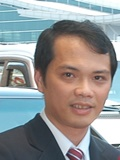Dr. Le Tuan Phuong Nam Position: Member of Division of Computational Mathematics and Engineering (CME), Institute for Computational Science (INCOS), Ton Duc Thang University, Ho Chi Minh City, Vietnam Areas of expertise: Computational Fluid Dynamics; Rarefied Gas Dynamics; Microfluidics and nanofluidics; Numerical methodsDr. Hoang Viet Long Position: Member of Division of Computational Mathematics and Engineering (CME), Institute for Computational Science (INCOS), Ton Duc Thang University, Ho Chi Minh City, Vietnam Areas of expertise: Stochastic analysis, Fuzzy analysis, Fuzzy Differential Equations; Fuzzy Partial Differential EquationsLe Anh Linh, PhD. Cadidate Position: Member of Division of Computational Mathematics and Engineering (CME), Institute for Computational Science (INCOS), Ton Duc Thang University, Ho Chi Minh City, Vietnam Areas of expertise: Numerical methods, structural reliability assessment, a reliability based design optimization for structures, artificial intelligenceLe The Hai, PhD. Cadidate Position: Member of Division of Computational Mathematics and Engineering (CME), Institute for Computational Science (INCOS), Ton Duc Thang University, Ho Chi Minh City, Vietnam Areas of expertise: Micro and Nano Systems TechnologyDr. Ao Hung Linh Position: Member of Division of Computational Mathematics and Engineering (CME), Institute for Computational Science (INCOS), Ton Duc Thang University, Ho Chi Minh City, Vietnam Areas of expertise: Fault diagnosis, signal processing, machine learning, optimization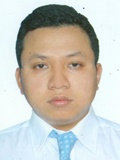Dr. Le Minh Triet Position: Member of Division of Computational Mathematics and Engineering (CME), Institute for Computational Science (INCOS), Ton Duc Thang University, Ho Chi Minh City, Vietnam Areas of expertise: Partial Differential Equation; Inverse problem; Functional Analysis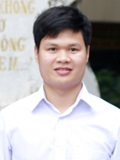Pham Toan Thang, PhD. Cadidate Position: Member of Division of Computational Mathematics and Engineering (CME), Institute for Computational Science (INCOS), Ton Duc Thang University, Ho Chi Minh City, Vietnam Areas of expertise: Mechanics of Composite Materials, Applied Mechanics, Solid Mechanics, Nonlinear Analysis of Mechanical Behavior of Materials and StructuresDr. Dang Tuan Hiep Position: Member of Division of Computational Mathematics and Engineering (CME), Institute for Computational Science (INCOS), Ton Duc Thang University, Ho Chi Minh City, Vietnam Areas of expertise: Algebraic Geometry, Computer AlgebraDr. Le Xuan Truong Position: Member of Division of Computational Mathematics and Engineering (CME), Institute for Computational Science (INCOS), Ton Duc Thang University, Ho Chi Minh City, Vietnam Areas of expertise: Ordinary differential equations; Partial differential equationsDr. Ho Vu Position: Member of Division of Computational Mathematics and Engineering (CME), Institute for Computational Science (INCOS), Ton Duc Thang University, Ho Chi Minh City, Vietnam Areas of expertise: Fuzzy differential equation, random fuzzy differential equation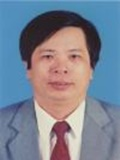Dr. Nguyen Dinh Phu Position: Member of Division of Computational Mathematics and Engineering (CME), Institute for Computational Science (INCOS),  Ho Chi Minh City, Ton Duc Thang University, Vietnam Areas of expertise: Stability Theory, Bifurcation Theory; System Theory; Sheaf-solutions of set Diferential Equations; Fuzzy Diferential Equations and Fuzzy Systems.; Set Diferential Equations and Set Control Systems; Interval - valued Diferential Equations and Interval - valued Control Equations; Stochastic Set Diferential Equations and Stochastic Set Control Systems; Fuzzy Random Diferential Equations and Fuzzy Random Integro - Diferential Equations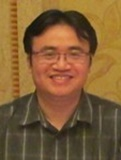Dr. Dinh Quang Hai Position: Member of Division of Computational Mathematics and Engineering (CME), Institute for Computational Science (INCOS), Ton Duc Thang University, Ho Chi Minh City, Vietnam Areas of expertise: Information Theory: Coding Theory, Algebra: Ring and Module Theory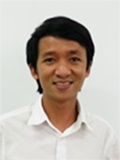Dr. Ta Quoc Bao Position: Member of Division of Computational Mathematics and Engineering (CME), Institute for Computational Science (INCOS), Ton Duc Thang University, Ho Chi Minh City, Vietnam Areas of expertise: Micro- and nano systems technologyDr. Mehran Mazandarani Position: Member of Division of Computational Mathematics and Engineering (CME), Institute for Computational Science (INCOS), Ton Duc Thang University, Ho Chi Minh City, Vietnam Areas of expertise: Fuzzy Logic System, Fuzzy Mathematics, Soft Computing, Fuzzy Modeling, Control Theory, Optimization, Intelligent and Conventional Control Dr. Mark Shattuck Position: Member of Division of Computational Mathematics and Engineering (CME), Institute for Computational Science (INCOS), Ton Duc Thang University, Ho Chi Minh City, Vietnam Areas of expertise: Enumerative and algebraic combinatorics; Discrete problems arising in pure and applied mathematics, physics, and computer science; Pattern avoidance; Decision theory; Recurrences and the kernel method; Euclidean geometryDr. Truong Chi Tin Position: Member of Division of Computational Mathematics and Engineering (CME), Institute for Computational Science (INCOS), Ton Duc Thang University, Ho Chi Minh City, Vietnam Areas of expertise: Stochastic optimization control; Artificial Intelligence; Data miningDr. Sergey Alexandrov Position: Member of Division of Computational Mathematics and Engineering (CME), Institute for Computational Science (INCOS), Ton Duc Thang University, Ho Chi Minh City, Vietnam Areas of expertise: Ideal Flow Theory; Singular Plastic Solutions Near Frictional Interfaces and Their Application to Fracture Prediction in Metal Forming; Fracture Analysis of Welded Structures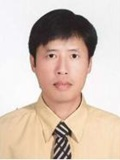Dr. Pham Tien Son Position: Member of Division of Computational Mathematics and Engineering (CME), Institute for Computational Science (INCOS), Ton Duc Thang University, Ho Chi Minh City, Vietnam Areas of expertise: Theory of singularities; Optimization of polynomial functions Dr. Nguyen Thi Thanh Lan Position: Member of Division of Computational Mathematics and Engineering (CME), Institute for Computational Science (INCOS), Ton Duc Thang University, Ho Chi Minh City, Vietnam Areas of expertise: Computation in social sciences; Mathematical analysis Dr. Le Phuong Position: Member of Division of Computational Mathematics and Engineering (CME), Institute for Computational Science (INCOS), Ton Duc Thang University, Ho Chi Minh City, Vietnam Areas of expertise: Qualitative properties of solutions to partial differential equations: existence, nonexistence, stability, symmetry and regularityDr. Pham Duy Khanh Position: Member of Division of Computational Mathematics and Engineering (CME), Institute for Computational Science (INCOS), Ton Duc Thang University, Ho Chi Minh City, Vietnam Areas of expertise: Optimization; Mathematical Programming; Variational Analysis

5. Former membersSon Nguyen Hoang, MSc Position: Former of Division of Computational Mathematics and Engineering (CME), Institute for Computational Science (INCOS), Ton Duc Thang University, Ho Chi Minh City, VietnamPhung Van Phuc, MSc Position: Former of Division of Computational Mathematics and Engineering (CME), Institute for Computational Science (INCOS), Ton Duc Thang University, Ho Chi Minh City, VietnamNguyen Thoi My Hanh, MSc Position: Former of Division of Computational Mathematics and Engineering (CME), Institute for Computational Science (INCOS), Ton Duc Thang University, Ho Chi Minh City, VietnamNguyen Minh Nhan, BSc Position: Former of Division of Computational Mathematics and Engineering (CME), Institute for Computational Science (INCOS), Ton Duc Thang University, Ho Chi Minh City, Vietnam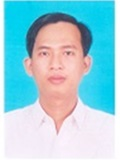Vo Ngoc Phu, MSc Position: Former of Division of Computational Mathematics and Engineering (CME), Institute for Computational Science (INCOS), Ton Duc Thang University, Ho Chi Minh City, VietnamDr. Phan Thanh Toan Position: Former of Division of Computational Mathematics and Engineering (CME), Institute for Computational Science (INCOS), Ton Duc Thang University, Ho Chi Minh City, Vietnam

6. Publications (ISI or Scopus only)

78. Khai Q. Le, Quang Minh Ngo; A silicon-on-insulator surface plasmon interferometer for hydrogen detection; Journal of Applied Physics, Volume 120 (2016), Issue 4, Article number 043106 (ISI).

77. Le Minh Triet, Luu Hong Phong; Regularization and error estimates for asymmetric backward nonhomogeneous heat equations in a ball; Electronic Journal of Differential Equations, Volume 2016 (2016), Article Number 256, pp. 1-12 (ISI).

76. Dinh Trung Hoa; Some inequalities for the matrix Heron mean; Linear Algebra and its Applications (2016) (ISI).

75. The Anh Bui, Xuan Thinh Duong, Xuan Truong Le; Regularity estimates for higher order elliptic systems on Reifenberg flat domains; Journal of Differential Equations, Volume 261 (2016), Pages 5637–5669 (ISI).

74. Le Xuan Truong, Le Cong Nhan; Existence of solutions for p-Laplacian-like differential equation with multi-point nonlinear Neumann boundary conditions at resonance; Electronic Journal of Differential Equations, Volume 2016 (2016), Article Number 206, Pages 1-17 (ISI).

73. T. Nguyen-Thoi, T. Rabczuk, V. Ho-Huu, L. Le-Anh, H. Dang-Trung, T. Vo-Duy; An Extended Cell-Based Smoothed Three-Node Mindlin Plate Element (XCS-MIN3) for Free Vibration Analysis of Cracked FGM Plates; International Journal of Computational Methods, Volume 14 (2016), Article Number 1750011 (ISI).

72. HungLinh Ao, T  Nguyen Thoi, V  Ho Huu, L Anh Le; A Support Vector Machine  Parameter Optimization Method Based on Backtracking Search Optimization Algorithm and Its Application for Roller Bearing Fault Diagnosis; International Journal of Acoustics and Vibration (ISI).

71. Thao Nguyen-Trang, Tai Vo-Van; A new approach for determining the prior probabilities in the classification problem by Bayesian method; Advances in Data Analysis and Classification, pp. 1-15 (ISI).

70. Nam T.P. Le, Ngoc Anh Vuc, Le Tan Loc; Effect of the sliding friction on heat transfer in high-speed rarefied gas flow simulations in CFD; International Journal of Thermal Sciences, Volume 109 (2016), Pages 334–341 (ISI).

69. T. Nguyen-Thoi, P. Phung-Van, M.H. Nguyen-Thoi, H. Dang-Trung; An upper-bound limit analysis of Mindlin plates using CS-DSG3 method and second-order cone programming; Journal of Computational and Applied Mathematics, Volume 281 (2015), Pages 32–48 (ISI).

68. Pham Toan Thang, T. Nguyen-Thoi; Effect of stiffeners on nonlinear buckling of cylindrical shells with functionally graded coatings under torsional load; Composite Structures, Volume 153 (2016), Pages 654–661 (ISI).

67. Pham-Toan Thang, T. Nguyen-Thoi; A new approach for nonlinear dynamic buckling of S-FGM toroidal shell segments with axial and circumferential stiffeners; Aerospace Science and Technology, Volume 53 (2016), Pages 1–9 (ISI).

66. Pham Toan Thang; Analytical solution for thermal buckling analysis of rectangular plates with functionally graded coatings; Aerospace Science and Technology, Vol 55 (2016), pp. 465 - 473 (ISI).

65. Nguyen Van Loi, Tran Dinh Ke, Valeri Obukhovskii, Pietro Zecca; Topological methods for some classes of differential variational inequalities; Journal of Nonlinear and Covnex Analysis, Volume 17 (2016), pp. 403-419 (ISI).

64. Thanh-Phong Dao; Multi-Response Optimization of a Compliant Guiding Mechanism Using Hybrid Taguchi-Grey Based Fuzzy Logic Approach; Mathematical Problems in Engineering, Volume 2016, Article ID 5386893 (ISI).

63. T. Vo-Duy, V. Ho-Huu, H. Dang-Trung, T. Nguyen-Thoi; A two-step approach for damage detection in laminated composite structures using modal strain energy method and an improved differential evolution algorithm; Composite Structures, Volume 147 (2016), pp. 42–53 (ISI).

62. Dinh Trung Hoa; An inequality for t–geometric means; Mathematical Inequalities & Applications, Vol 19 (2016), Number 2, pp. 765–768 (ISI).

61. Pham-Toan Thang, T. Nguyen-Thoi*, Jaehong Lee; “Closed-form expression for nonlinear analysis of imperfect sigmoid-FGM plates with variable thickness resting on elastic medium”, Composite Structures, Volume 143 (2016), pp. 143-150 (ISI).

60. V. Ho-Huu, T. Nguyen-Thoi, T. Vo-Duy, T. Nguyen-Trang, “An adaptive elitist differential evolution for optimization of truss structures with discrete design variables”, Computers and Structures, 165 (2016), pp. 59-75 (ISI).

59. Thanh-Phong Dao and Shyh-Chour Huang, “Design, fabrication, and predictive model of a 1-dof translational flexible bearing for high precision mechanism”, Transactions of the Canadian Society for Mechanical Engineering, Volume 39 (2015), No. 3, pp. 419 - 429 (ISI).

58. Ngo Van Hoa, “Fuzzy fractional functional integral and differential equations”, Fuzzy Sets and Systems (2015), Volume 280, pp. 58-90 (ISI).

57. Sy Dzung Nguyen, Quoc Hung Nguyen, “Design of active suspension controller for train cars based on sliding mode control, uncertainty observer and neuro-fuzzy system”, Journal of Vibration and Control (2015), DOI: 10.1177/1077546315592767 (ISI).

56. Ngo Van Hoa, “The initial value problem for interval-valued second-order differential equations under generalized H-differentiability”, Information Sciences, Volume 311 pages 119-148 (2015) (ISI).

55. Ho Vu, Le Si Dong, “Initial value problem for second-order random fuzzy differential equations”, Advances in Difference Equations, 2015:373 (2015) (ISI).

54. Hai Le The, Bao Quoc Ta*, Hoa Le Thanh, Tao Dong, Trung Nguyen Thoi & Frank Karlsen, “Geometric effects on mixing performance in a novel passive micromixer with trapezoidal-zigzag channels”, Journal of Micromechanics and Microengineering, 25 , 094004, 11pp (2015) (ISI).

53. Dinh Trung Hoa, “On characterization of operator monotone functions”, Linear Algebra and its Applications, 487, 260 - 267 (2015) (ISI).

52. Nguyen Van Loi, Valeri Obukhovskii, Jen-Chih Yao, “A multiparameter global bifurcation theorem with application to a feedback control system”, Fixed Point Theory, 16, No. 2, 353-370 (2015) (ISI).

51. Truong Khang Nguyen and Ikmo Park (2015), “Design of a Substrate-Integrated Fabry-Perot Cavity Antenna for K-Band Applications”, International Journal of Antennas and Propagation, Volume 2015, Article ID 73801, 12 pages (2015) (ISI).

50. Hiep Nguyen Duc, Kelly Rivett, Katrina MacSween & Linh Le-Anh, “Association of climate drivers with rainfall in New South Wales, Australia, using Bayesian Model Averaging”, Theoretical and Applied Climatology, DOI 10.1007/s00704-015-1622-8 Vol 121, 7-17 (2015) (ISI).

49. Dinh Trung Hoa , Vo Thi Bich Khue & Hiroyuki Osaka, “A generalized reverse Cauchy inequality for matrices”, Linear and Multilinear Algebra, DOI:10.1080/03081087.2015.1087459 (2015) (ISI).

48. Nam T.P. le , Ehsan Roohi, “A new form of the second-order temperature jump boundary condition for the low-speed nanoscale and hypersonic rarefied gas flow simulations”, International Journal of Thermal Sciences, 98, 51 - 59 (2015) (ISI).

47. Dinh Trung Hoa , Osaka H. and Ho Minh Toan, “Interpolation classes and matrix means”, Banach Journal of Mathematical Analysis, 9 (2015), no. 3, 140–152 (2015) (ISI).

46. P. Phung-Van, T. Nguyen-Thoi, T. Bui-Xuan, Q. Lieu-Xuan, “A cell-based smoothed three-node Mindlin plate element (CS-FEM-MIN3) based on the C0-type higher-order shear deformation for geometrically nonlinear analysis of laminated composite plates”, Computational Materials Science, 96 (Part B): 549 - 558 (2015) (ISI).

45. Vasile Lupulescu , Le Si Dong, Ngo Van Hoa*, “Existence and uniqueness of solutions for random fuzzy fractional integral and differential equations”, Journal of Intelligent and Fuzzy Systems, 29 (2015), 27-42 (2015) (ISI).

44. M.H. Nguyen-Thoi, L. Le-Anh, V. Ho-Huu, T. Nguyen-Thoi, “An extended cell-based smoothed discrete shear gap method (XCS-FEM-DSG3) for free vibration analysis of cracked Reissner-Mindlin shells”. Frontiers of Structural and Civil Engineering (2015) (ISI).

43. T. Vo-Duy, N. Nguyen-Minh, H. Dang-Trung, A. Tran-Viet, T. Nguyen-Thoi; “Damage assessment of laminated composite beam structures using damage locating vector (DLV) method”; Frontiers of Structural and Civil Engineering, Volume 9, pp 457-465 (2015) (Scopus).

42. Truong Khang Nguyen, Quoc Bao Ta, and Ikmo Park, “Design of a Planar, High-Gain, Substrate-Integrated Fabry-Perot Cavity Antenna at Terahertz Frequency”, Current Applied Physics, Vol 15, pp 1047-1053 (2015) (ISI).

41. N. Nguyen-Minh, T. Nguyen-Thoi, T. Bui-Xuan, T. Vo-Duy, “Static and free vibration analyses of stiffened folded plates using a cell-based smoothed discrete shear gap method (CS-FEM-DSG3)”, Applied Mathematics and Computation, Vol 266, 212–234 (2015) (ISI).

40. Hoa Le Thanh, Bao Quoc Ta*, Hai Le The, Vy Nguyen, Kaiying Wang and Frank Karlsen, "Low-cost Fabrication of Hollow Microneedle Arrays Using CNC machining and UV Lithography", Journal of Microelectromechanical Systems, Doi: 10.1109/JMEMS.2015.2424926 (2015) (ISI).

39. L. Le-Anh, T. Nguyen-Thoi, V. Ho-Huu, H. Dang-Trung, T. Bui-Xuan, “Static and frequency optimization of folded laminated composite plates using an adjusted differential evolution algorithm and a smoothed triangular plate element”, Composite Structures; 127: 382-394 (2015) (ISI).

38. Khader M. Hamdia, Tom Lahmer, Trung Nguyen-Thoi, Timon Rabczuk, “Predicting The Fracture Toughness of PNCs: A Stochastic Approach Based on ANN and ANFIS”, Computational Materials Science; 102: 304-313 (2015) (ISI).

37. Truong Khac Tung, Kenli Li, Yuming Xu, Aijia Ouyang, Tien Trong Nguyen, “Solving 0-1 knapsack problem by artificial chemical reaction optimization algorithm with a greedy strategy”, Journal of Intelligent and Fuzzy Systems. DOI:10.3233/IFS-141500. 2179–2186 (2015) (ISI).

36. Tran Van Thuy, YaoNan Wang, Ao Hung Linh, Truong Khac Tung, “Sliding Mode Control Based on Chemical Reaction Optimization and Radial Basis Functional Link Net for De-Icing Robot Manipulator”, Journal Of Dynamic Systems Measurement And Control-Transactions Of The Asme , Vol 137, Issue 5, DOI:10.1115/1.4028886 Paper No: DS-14-1180 (2015) (ISI).

35. Nguyen Van Loi, Mai Quoc Vu, Pham Tuan Cuong, "Nonlocal problem for differential complementarity systems", Applied Mathematics and Computation, 265(2015), 799-806 (2015) (ISI).

34. Xiaohui Fua & Dinh Trung Hoa*, “On some inequalities with matrix means”, Linear and Multilinear Algebra (2015) (ISI).

33. V. Ho-Huu, T. Nguyen-Thoi, M.H. Nguyen-Thoi, L. Le-Anh, “An improved constrained differential evolution using discrete variables (D-ICDE) for layout optimization of truss structures”. Expert Systems With Applications, Vol 42, Issue 20, 7057 - 7069 (2015) (ISI).

32. Songrong Luo, Junsheng Cheng, and HungLinh Ao, “Application of LCD-SVD Technique and CRO-SVM Method to Fault Diagnosis for Roller Bearing” Shock and Vibration, Vol 2015, Article ID 847802 (2015) (ISI).

31. Nguyen Van Loi, Zhenhai Liu and Valeri Obukhovskii, “On an a-bifurcation theorem with application to a parameterized integro-differential system”, Fixed Point Theory, 16(2015), No. 1, 127-142 (2015) (ISI).

30. Dinh Trung Hoa & Hiroyuki Osaka, “Inequalities for interpolation functions”, Banach Journal of Mathematical Analysis, doi:10.15352/bjma/09-1-6, Vol. 9, No. 1, 67-74 (2015) (ISI).

29. T. Nguyen-Thoi, P. Phung-Van, V. Ho-Huu, L. Le-Anh, “An edge-based smoothed finite element method (ES-FEM) for dynamic analyses of 2D fluid-solid interaction problems”, KSCE Journal of Civil Engineering; Vol 19, Issue 3, 641-650.

28. Nam T.P. Le, Ahmad Shoja-Sani, Ehsan Roohi, “Rarefied gas flow simulations of NACA 0012 airfoil and sharp 25–55-deg biconic subject to high order nonequilibrium boundary conditions in CFD”, Aerospace Science and Technology, 274–288.

27. Hai Le The, Hoa Le Thanh, Tao Dong, Bao Quoc Ta, Nhut Tran-Minh, and Frank Karlsen, “An Effective Passive Micromixer with Shifted Trapezoidal Blades Using Wide Reynolds Number Range”, Chemical Engineering Research and Design, DOI:10.1016/j.cherd.2014.12.003, Vol 93, 1–11.

26. Ngo Van Hoa, “Fuzzy fractional functional differential equations under Caputo gH-differentiability”, Communications in Nonlinear Science and Numerical Simulation, Volume 22, Issues 1–3, Pages 1134–1157 (2015) (ISI).

25. Vu-H, Dong. S-Le and Phu. D-Nguyen, “On Random Set-Valued Functional Integro Differential Equations With The Second Type Hukuhara Derivative” Dynamics of Continuous, Discrete and Impulsive Systems Series A: Mathematical Analysis 21, 267-289.

24. Ho Vu, S. Sivasundaram, “On the solution of set random dynamic equations on time scales”, Nonlinear Studies, Vol. 21, No. 4, pp. 703-719.

23. Dong. S-Le, Vu-H, Phu. D-Nguyen, “The formulas of the solution for linear-order random fuzzy differential equations”, Journal of Intelligent and Fuzzy Systems. DOI:10.3233/IFS-141362.

22. Nguyen Dinh Phu, Truong Vinh An, Ngo Van Hoa, Nguyen Thi Hien, “Interval-valued functional differential equations under dissipative conditions”, Advances in Difference Equations 2014:198. DOI:10.1186/1687-1847-2014-198 (2014) (ISI).

21. Dinh Trung Hoaab, Hiroyuki Osakac and Jun Tomiyamad, “Characterization of operator monotone functions by Powers–Størmer type inequalities”, Taylor & Francis, DOI: 10.1080/03081087.2014.954571& (2014) (ISI).

20. H. Nguyen-Van, N. Nguyen-Hoai, T. Chau-Dinh, T. Nguyen-Thoi, “Geometrically nonlinear analysis of composite plate and shell via a quadrilateral element with good coarse-mesh accuracy”, Composite Structures, 112:327–338 (2014) (ISI).

19. T. Nguyen-Thoi, T. Rabczuk, T. Lam-Phat, V. Ho-Huu, P. Phung-Van, “Free vibration analysis of cracked Mindlin plate using an extended cell-based smoothed discrete shear gap method (XCS-DSG3)”, Theoretical and Applied Fracture Mechanics; 72: 150-163 (2014) (ISI).

18. Ngo Van Hoa, Phan Van Tri, Tran Trong Dao, Ivan Zelinka, “Some global existence results and stability theorem for fuzzy functional differential equations”, Journal of Intelligent and Fuzzy Systems, DOI 10.3233/IFS-141315 (2014) (ISI).

17. T. Nguyen-Thoi, P. Phung-Van, S. Nguyen-Hoang, Q. Lieu-Xuan, “A coupled alpha-FEM for dynamic analyses of 2D fluid-solid interaction problems”, Journal of Computational and Applied Mathematics, 271: 130 - 149 (2014) (ISI).

16. Truong Vinh An, Nguyen Dinh Phu, Ngo Van Hoa, “A note on solutions of interval-valued Volterra integral equations”, Journal of Integral Equations and Applications, Volume 26, Number 1 (2014) (ISI).

15. Ngo Van Hoa, Nguyen Dinh Phu, Tran Thanh Tung, Le Thanh Quang, “Interval-valued functional integro-differential equations”, Advances in Difference Equations 2014:177. DOI:10.1186/1687-1847-2014-177 (2014) (ISI).

14. T. Nguyen-Thoi, T. Bui-Xuan, P. Phung-Van, S. Nguyen-Hoang, H. Nguyen-Xuan, “An edge-based smoothed three-node Mindlin plate element (ES-MIN3) for static and free vibration analyses of plates”, KSCE Journal of Civil Engineering, 18 (4): 1072 - 1082 (2014) (ISI).

13. P. Phung-Van, H. Luong-Van, T. Nguyen-Thoi, H. Nguyen-Xuan, “A cell-based smoothed discrete shear gap method (CS-DSG3) based on the higher-order shear deformation theory for dynamic responses of Mindlin plates on the viscoelastic foundation subjected to a moving sprung vehicle”, International Journal for Numerical Methods in Engineering, 98 (13), 988-1014 (2014) (ISI).

12. Vu-H, Dong. S-Le, Hoa. V-Ngo, “Random fuzzy functional integro-differential equations under generalized Hukuhara differentiability” Journal of Intelligent and Fuzzy Systems, Doi: 10.3233/IFS-131116 (2014) (ISI).

11. Phan Van Tri, Ngo Van hoa, Nguyen Dinh Phu, “Sheaf fuzzy problems for functional differantial equations”, Advances in Difference Equations (2014) (ISI).

10. Ho Vu, Ngo Van Hoa, Nguyen Dinh Phu, “The local existence of solutions for random fuzzy integro-differential equations under generalized H-differentiability”, Journal of Intelligent and Fuzzy Systems, DOI 10.3233/IFS-130940(2014) (ISI).

9. P. Phung-Van, T. Nguyen-Thoi, H. Dang-Trung, N. Nguyen-Minh, “A cell-based smoothed discrete shear gap method (CS-FEM-DSG3) using layerwise theory based on the C0-type higher-order shear deformation for static and free vibration analyses of sandwich and composite plates”, Composite Structures, 111: 553-565 (2014) (ISI).

8. T. Nguyen-Thoi, P. Phung-Van, S. Nguyen-Hoang, Q. Lieu-Xuan, “A smoothed coupled NS/nES-FEM for dynamic analysis of 2D fluid-solid interaction problems”, Applied Mathematics and Computation, 232: 324-346 (2014) (ISI).

7. P. Phung-Van, T. Nguyen-Thoi, H. Luong-Van, C. Thai-Hoang, H. Nguyen-Xuan, “A cell-based smoothed discrete shear gap method (CS-FEM-DSG3) using layerwise deformation theory for dynamic response of composite plates resting on viscoelastic foundation”, Computer Methods in Applied Mechanics and Engineering, 272: 138-159 (2014) (ISI).

6. P. Phung-Van, Chien H. Thai, T. Nguyen-Thoi, H. Nguyen-Xuan, “Static and free vibration analyses of composite and sandwich plates by an edge-based smoothed discrete shear gap method (ES-DSG3) using triangular elements based on layerwise theory”, Composites Part B: Engineering, 60: 227-238 (2014) (ISI).

5. P. Phung-Van, T. Nguyen-Thoi, H. Luong-Van, Q. Lieu-Xuan, “Geometrically nonlinear analysis of functionally graded plates using a cell-based smoothed three-node plate element (CS-MIN3) based on the C0-HSDT”, Computer Methods in Applied Mechanics and Engineering, 270: 15-36 (2014) (ISI).

4. H. Luong-Van, T. Nguyen-Thoi, GR. Liu, P. Phung-Van, “A cell-based smoothed finite element method using three-node shear-locking free Mindlin plate element (CS-FEM-MIN3) for dynamic response of laminated composite plates on viscoelastic foundation”, Engineering Analysis with Boundary Elements, 42: 8-19 (2014) (ISI).

3. Ngo Van Hoa, Nguyen Dinh Phu, “Global existence of solutions for interval-valued second-order differential equations under generalized Hukuhara derivative”, Advances in Difference Equations 2013, 2013:290 doi:10.1186/1687-1847-2013-290.

2. Truong Vinh An, Ngo Van Hoa, Nguyen Dinh Phu, “Global existence of solutions for interval-valued integro-differential equations under generalized H-differentiability”, Advances in Difference Equations, 2013:217 doi:10.1186/1687-1847-2013-217(2013) (ISI).

1. P. Phung-Van, T. Nguyen-Thoi, T. Le-Dinh, H. Nguyen-Xuan, “Static, free vibration analyses and dynamic control of composite plates integrated with piezoelectric sensors and actuators by the cell-based smoothed discrete shear gap method (CS-FEM-DSG3)”, Smart Materials and Structures, 22 (9): 095026 (2013) (ISI).